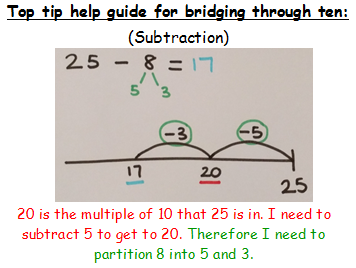### Bridging Round and Adjust (Subtraction)

To help us count over tens we can use a method called bridging. This is when we bridge through the multiples of ten when we are adding or subtracting a number that will take us over the tens boundary. Here is an example of bridging for subtraction.

Remember: When we subtract we move from right to left on our number line because our numbers are decreasing in value. When we add on a number line we move from left to right because the value of our numbers are increasing!